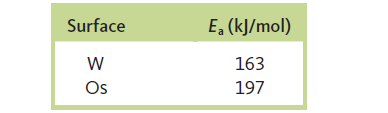# Problem: The decomposition of NH3 to N2 and H2 was studied on two surfaces:Without a catalyst, the activation energy is 335 kJ/mol.a. Which surface is the better heterogeneous catalyst for the decomposition of NH3? Why?b. How many times faster is the reaction at 298 K on the W surface compared with the reaction with no catalyst present? Assume that the frequency factor A is the same for each reaction.c. The decomposition reaction on the two surfaces obeys a rate law of the formRate = k [NH3] / [H2]How can you explain the inverse dependence of the rate on the H2 concentration?

###### FREE Expert Solution

The decomposition reaction on the two surfaces obeys a rate law of the form

Rate = k [NH3] / [H2]

The decomposition reaction is an equilibrium reaction:

2 NH3 ⇌ N2 + 3 H2

89% (403 ratings)###### Problem Details

The decomposition of NH3 to N2 and H2 was studied on two surfaces:Without a catalyst, the activation energy is 335 kJ/mol.

a. Which surface is the better heterogeneous catalyst for the decomposition of NH3? Why?
b. How many times faster is the reaction at 298 K on the W surface compared with the reaction with no catalyst present? Assume that the frequency factor A is the same for each reaction.

c. The decomposition reaction on the two surfaces obeys a rate law of the form

Rate = k [NH3] / [H2]

How can you explain the inverse dependence of the rate on the H2 concentration?

Frequently Asked Questions

What scientific concept do you need to know in order to solve this problem?

Our tutors have indicated that to solve this problem you will need to apply the Arrhenius Equation concept. You can view video lessons to learn Arrhenius Equation. Or if you need more Arrhenius Equation practice, you can also practice Arrhenius Equation practice problems.

What professor is this problem relevant for?

Based on our data, we think this problem is relevant for Professor Millhauser's class at UCSC.

What textbook is this problem found in?

Our data indicates that this problem or a close variation was asked in Chemistry: An Atoms First Approach - Zumdahl Atoms 1st 2nd Edition. You can also practice Chemistry: An Atoms First Approach - Zumdahl Atoms 1st 2nd Edition practice problems.